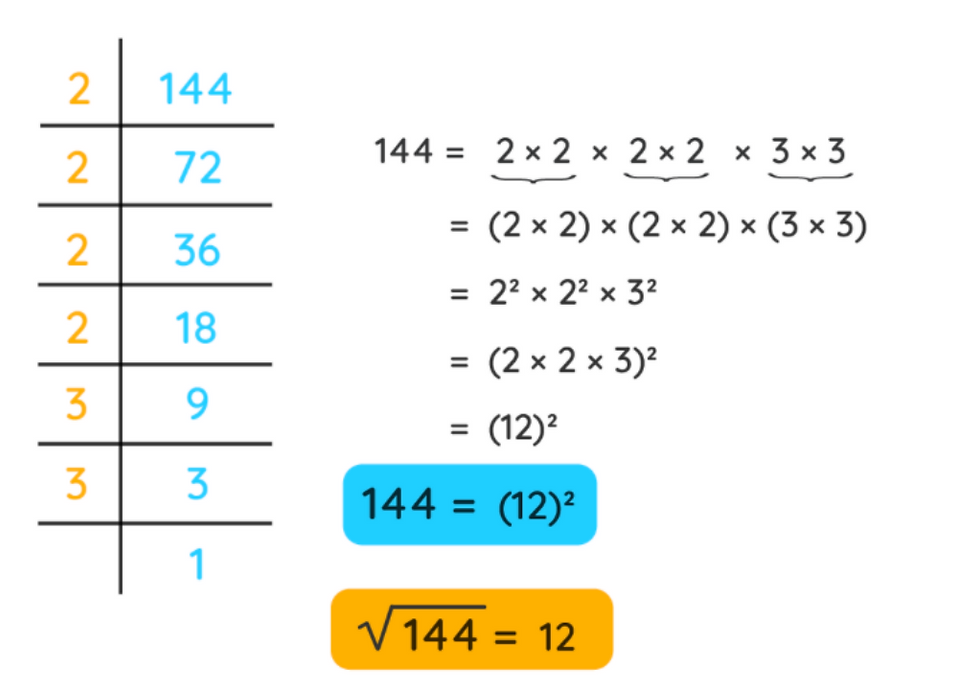top of page

# CSEET Quantitative aptitude - square rootSquare Root

The square root of a number is the inverse operation of squaring a number. The square of a number is the value that is obtained when we multiply the number by itself, while the square root of a number is obtained by finding a number that when squared gives the original number.

If 'a' is the square root of 'b', it means that a × a = b. The square of any number is always a positive number, so every number has two square roots, one of a positive value, and one of a negative value. For example, both 2 and -2 are square roots of 4. However, in most places, only the positive value is written as the square root of a number.

What is Square Root?

The square root of a number is that factor of a number which when multiplied by itself gives the original number. Squares and square roots are special exponents. Consider the number 9. When 3 is multiplied by itself, it gives 9 as the product. This can be written as 3 × 3 or 32. Here, the exponent is 2, and we call it a square. Now when the exponent is 1/2, it refers to the square root of the number. For example, √n = n1/2, where n is a positive integer.

Square Root Definition

The square root of a number is the value of power 1/2 of that number. In other words, it is the number whose product by itself gives the original number. It is represented using the symbol '√ '. The square root symbol is called a radical, whereas the number under the square root symbol is called the radicand.

How to Find Square Root?

To find the square root of a number, we just see by squaring which number would give the actual number. It is very easy to find the square root of a number that is a perfect square. Perfect squares are those positive numbers that can be expressed as the product of a number by itself. In other words, perfect squares are numbers which are expressed as the value of power 2 of any integer. We can use four methods to find the square root of numbers and those methods are as follows:

Repeated Subtraction Method

Prime Factorization Method

Estimation Method

Long Division Method

It should be noted that the first three methods can be conveniently used for perfect squares, while the fourth method, i.e., the long division method can be used for any number whether it is a perfect square or not.

Repeated Subtraction Method of Square Root

This is a very simple method. We subtract the consecutive odd numbers from the number for which we are finding the square root, till we reach 0. The number of times we subtract is the square root of the given number. This method works only for perfect square numbers. Let us find the square root of 16 using this method.

16 - 1 = 15

15 - 3 =12

12 - 5 = 7

7- 7 = 0

You can observe that we have subtracted 4 times. Thus,√16 = 4

Square Root by Prime Factorization Method

Prime factorization of any number means to represent that number as a product of prime numbers. To find the square root of a given number through the prime factorization method, we follow the steps given below:

Step 1: Divide the given number into its prime factors.

Step 2: Form pairs of factors such that both factors in each pair are equal.

Step 3: Take one factor from the pair.

Step 4: Find the product of the factors obtained by taking one factor from each pair.

Step 5: That product is the square root of the given number.

Let us find the square root of 144